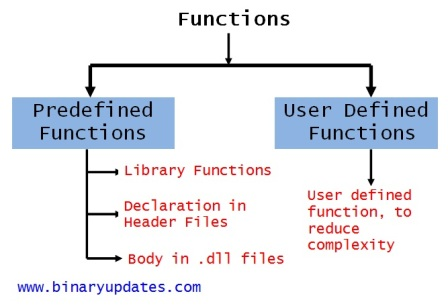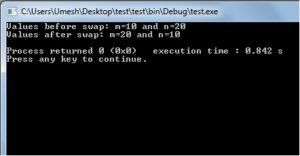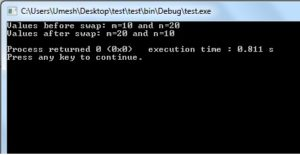Friday , August 23 2019

# Functions in C Programming

Functions in C Programming are building block of a Program. A function is a block of code that performs a specific task.  There are times when we need to write particular block of code more than once in our program. This may increase complexity of a program. To make programmers life easy C Programming support functions which allows programmer to declare and define a group of statements once and that can be called and used whenever required.

#### Introduction of Functions in C

Dividing complex problem into small components makes program easy to understand and use. There are two types of functions Standard Library Functions and User Defined Functions.Types of Functions in C
##### Library Functions

The functions which are defined by C library example printf(), scanf(), strcat() etc. You just need to include appropriate header files to use these functions. These are already declared and defined in C libraries.

##### User-defined Functions

The functions which are defined by the user at the time of writing program. Functions are made for code reusability and for saving time and space. Functions that a programmer writes will generally require a prototype. Just like a blueprint, the prototype gives basic structural information: it tells the compiler what the function will return, when the function will be called, as well as what arguments the function can be passed. When I say that the function returns a value, I mean that the function can be used in the same manner as a variable would be.

##### Example: Simple Functions

Let’s have a look at simple example and then we will break down program to understand.

Explanation: There can be more than one argument passed to a function or none at all (where the parentheses are empty), and it does not have to return a value. Functions that do not return values have a return type of void.

In this example, int add (int x, int y); is function prototype. This prototype specifies that the function add will accept two arguments, both integers, and that it will return an integer. Do not forget semi-colon while writing function prototype. Without it, the compiler will probably think that you are trying to write the actual definition of the function. Now let’s look at actual function definition:

The add function takes two integer numbers in terms of x and y. return is keyword used to force the function to return an integer value and so we have defined function add as type integer. In main routine most of statements are self explanatory but still we’ll look at:

In this statement we have called add function by calling it into printf statement. The beauty of function is we can use add function as many time as we want in the program. So we need not to write logic all over again. This certainly allows us to reuse code.

#### Why Need Functions?

The function can be used for many reasons, say for example programmer writes a block of code that he wants to use 20-30 times throughout the program. A function to execute that code would save lots of space and time, at same time makes program more readable. As we define function only once, which makes it easy to modify at one place and change reflects all over the places. The functions in C programs also allowed us to break down complex program into logical parts. For example, take calculator program that runs a complex code with variety of different functions like addition, subtraction, multiplication, division and so on. The best way is to break down complex calculator program into smaller and manageable tasks in the form of function. Each task like addition, subtraction, multiplication and division has been defined in its own function which makes a sense while reading and modifying code.

There are two ways that a C function can be called from a program. They are,

• Call by Value
• Call by Reference

#### Call by Value in C Programming

In call by value method, the value of the variable is passed to the function as parameter. The value of the actual parameter can’t be modified by formal parameter. Different Memory is allocated for both actual and formal parameters, because value of actual parameter is copied to formal parameter. Note:

• Actual parameter – This is the argument which is used in function call
• Formal parameter – This is the argument which is used in function definition
##### Example: Function using call by value
Explanation: In this program, the values of the variables “m” and “n” are passed to the function “swap”. These values are copied to formal parameters “a” and “b” in swap function and used.Output of Call by Value Program

#### Call by Reference in C Programming

In call by reference method, the address of the variable is passed to the function as parameter. The value of the actual parameter can be modified by formal parameter. Same memory is used for both actual and formal parameters since only address is used by both parameters.

Example: Function using Call by Reference

Explanation: In above program, the address of the variables “m” and “n” are passed to the function “swap”. These values are not copied to formal parameters “a” and “b” in swap function, because they are just holding the address of those variables. This address is used to access and change the values of the variables.Output of Call by Reference Program

This may be difficult to understand for newbie’s if you’re not familiar with pointers in C language. We’ll cover pointers in our future lesson. So don’t worry if you feel difficult to understand for this moment.

Now we know how to create user defined Functions in C Programming. In next post, we’ll learn about Recursion in C Programming. Please do write us if you have any suggestion/comment or come across any error on this page. Thanks for reading!

### About Umesh LokhandeUmesh Lokhande holds a Master degree in Scientific Instrumentation from University of Applied Sciences Jena, Germany. and has previously worked at Orbotech, Alere Technologies etc. Umesh is also a founder and first author of BINARYUPDATES.COM